# Mathematics 1010 online

## Irrational Numbers

Consider a right triangle whose two short sides have a length of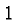foot each. By the Pythagorean Theorem the long side has a length of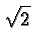feet. It turns out thatis not a rational number .

This remarkable fact can be seen by a classic argument usually attributed to Eudoxus of Cnidus (approx. 406-355BC). We assume thatis in fact rational and then derive a contradiction. Since there are no contradictions in mathematics our assumption must be false and somust be irrational.

So let us suppose thatcan be written as a ratio of two integers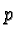and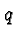: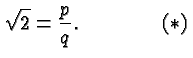We don't knowand, and if they exist they may be very large. However, we may assume thatandhave no factors greater thanin common, because if they did we could cancel that factor in the expression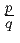. In particular, we may assume thatandare not both even. (One may be, or the other, but not both.)

We start with the equation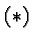. Squaring on both sides gives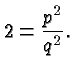Multiplying with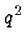on both sides gives the new equation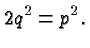Because of the factor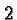on the left side, the right side, i.e.,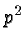, must be even. For this to be true,itself must be even. Ifis even thenis divisible by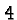. Hence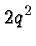is also divisible bywhich means thatmust be even. That implies in turn thatmust be even. Thusandmust be both even, which contradicts our (legitimate) assumption thatandhave no factor in common. Our (doubtful) assumption thatis rational therefore can't be true -- the square root ofis not a rational number.

The following facts regarding irrational numbers are beyond the scope of this class, but you can read about them in the book "What is Mathematics" by Courant Robbins, or I would be pleased to tell you more if you are interested:

• The square roots of prime numbers are irrational.

•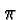is irrational.

• A rational number can be written as a repeating (or terminating) decimal.

• A repeating or (or terminating) decimal can be written as a fraction of two integers, i./e., it's rational.

• In a well defined sense, most real numbers are irrational. In other words, there are more irrational numbers than rational numbers.

• In the same sense there are as many (but in particular no more) rational numbers than there are integers. This is true despite the fact that all integers are rational, and some rational numbers aren't integers. Infinity is different!